# Data for AI in Health Care

How can data be used to answer research questions or define a Machine Learning problem and propose a solution to improve the health of patients and population.

A data mining workflow as shown below will help us answer the above question. We will walk through an example using the data mining workflow.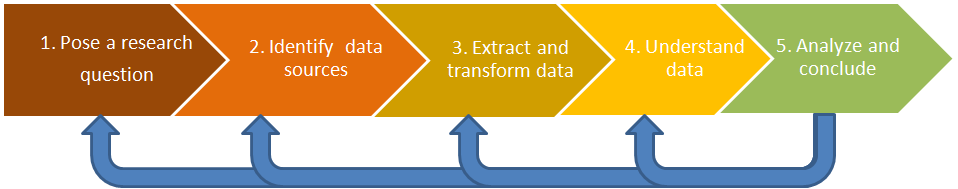Fig 1. Data Mining Workflow

# Step 1 — Research Question

What factors contributing to health care expenditures will help identify what sort of programs to implement to reduce future expenditures for the elderly.

# Step 2 — Data Source

The data comes from the 2005 Medical Expenditures Panel Survey. We explore a total of 18 variables that impact the total expanse of health care among the elderly.

# Step 3 — Extract and transform data

Extracted the data from a cvs file and stored the data in two data frames for analysis.

# Step 4 — Understanding the Data

Is the data normally distributed? Do you need to transform the data for analysis? Is there correlation amongst the predictor variables? These are some of the basic questions, one needs to answer.

## Exploring the Response Variable: Total Expense

The total expense is right-skewed, as shown in Fig 2. Using a log transformation on the variable provided a more normal distribution.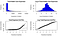Fig. 2 — Response Variable — Total Expense

## Exploring the predictor variables

A quick review of the correlation of the data indicates that multicollinearity may not be an issue, as all correlations are less than 0.5, which we will validate through the variance inflation factor (VIF).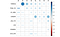Fig. 3 — Correlation

# Step 5 — Analyze and Conclusions

You can run the data through multiple regression models and ranked them by measuring the Root Mean Squared Errors, the R-Square and the Accuracy of the models, to select the best model.

We started with standard linear regression model. We checked for multicollinearity utilizing variance inflation factor (VIF). We noticed that the VIF (Table 1) for all the variables was less than 2, indicating that there is no correlation between the predictor variables.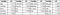Table 1 — VIF for predicator variables

Next we tested the model for heteroscedasticity utilizing the non-constant variance test (ncvTest) and also plotting the standardized residuals to see if they displayed a statistically normal distribution, (Fig. 4), indicating that the errors have the same but unknown variance. The ncvTest indicated that the residuals showed heteroscedasticity.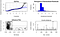Fig. 4— Initial regression model

To address the heteroscedasticity nature of the model, we ran a new model by applying a logarithmic transformation of the response variable ‘totalexpense’ (Fig. 5).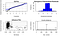Fig. 5— Log transformation

## Basic Regression

We generated a baseline by running the basic regression model with all the predictor variables. We used the results to spot any problems that need to be addressed. The initial model indicates that only 4 out of the 18 predictor variables are significant. The adjusted R-square for the model is 0.536.

## Regression with Log transformation

To answer the heteroscedasticity nature of the basic regression model, we applied a log transformation on the response variable. This model indicates that 8 out of the 18 predictor variables are significant. The adjusted R-square for the model is 0.482.

The histogram of the residuals indicates a close to normal distribution (Fig. 5). But the Anderson-Darling normality test returned a p-value of 2e-5 suggesting that we reject the null hypothesis that the residuals are normally distributed.

## Ridge, Lasso and Elastic Regression

You can run the data through ridge, lasso and elastic regression utilizing the glmnet package.

## Ranking and Selecting the model

You should then rank and select the best model for your data that answers your research question.

We used cross-validation with n-folds equal to 10, to see how well the various models predict and ranked them by measuring the Root Mean Squared Errors, the R-Square and the Accuracy of the models.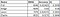Fig 6 — Basic Data Model

If we want to select a model with the best accuracy, then we select the Ridge model.

## Conclusion

All the models displayed heteroscedastic nature of residuals. Heteroscedasticity is a problem because ordinary least squares (OLS) regression assumes that all residuals are drawn from a population that has a constant variance (homoscedasticity). To satisfy the regression assumptions and be able to trust the results, the residuals should have a constant variance. This could be due to the large range between the largest and the smallest observed values. The response variable (totalexp) has smallest value of 58 and largest value of 107355. One of the predictor variable (income) has smallest value of 125 and largest value of 176839.

We think other predictor variables should be explored to better understand the cost of health care amongst the elders.

Where you able to answer your research question? Are you happy with the accuracy of your model? Do you want to explore other predictor variables? Do you need more data?

Based on our results we will go back to identifying a better data source that would answer our research question and go through the steps outlined in the data mining workflow, till we are happy with our results.